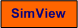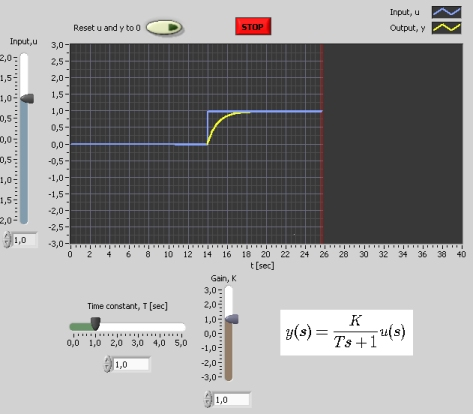# Time Constant

Snapshot of the front panel of the simulator:• What is needed to run the the simulator? Read to get most recent information!
• Tips for using the simulator.
• The simulator: . The simulator runs immediately after the download by clicking Open in the download window. Alternatively, you can first save a copy of the exe-file on any directory (folder) on your PC and then run the exe-file, which starts the simulator.

## Description of the system to be simulated

In this lab the step response of a general or standard first order system is simulated (that is, the time response on the output of the system is calculated numerically).

A mathematical model of the first order system is the following differential equation:

T dy(t)/dt + y(t) = Ku(t)    (Eq. 1)

where

• K is the gain
• T is the time-constant

An alternative way of representing this model is by Laplace transforming the differential equation, and taking the ratio of output to input (in the Laplace domain), to get the transfer function from input u to output y:

h(s) = y(s)/u(s) = K/(Ts+1)    (Eq. 2)

## Aim of this lab

The aim is to develop both a qualitative and a quantitative understanding of the impact that the gain K and the time-constant T, and the step input height U have on the step respons of a first order system.

## Motivation

First order systems constitute an important class of dynamic systems: Many physical systems behave (approximately) as first order systems, e.g. stirred liquid tanks, motors, and sensors. And a very common lowpass filter algorithm is a first order system.

Note: The equality sign "=" in the following text can be regarded as "approximately equal to" (so that you do not have to enter exact numeric values).

1. Impact of gain K: Set U=2 and T=1. Adjust K.
1. What is the impact of K on the stationary response?
2. What is the impact of K on the response-time?

2. Impact of time-constant T: Unless otherwise instructed, set U=2 and K=2. Adjust T.
1. What is the impact of T on the stationary response?
2. What is the impact of T on the response-time?
3. Adjust U up and down continuously (like a sinusoidal variation) for a small and for a large value of T. How does T influate the "speed" of the system?

3. Impact of step height U: Set K=2 and T=1. Adjust U.
1. What is the impact of T on the stationary response?
2. What is the impact of T on the response-time?

4. Very large time-constant: Set U=1, T=100, and K=100 (enter these values into the respective numeric displays). Characterize the step response. Explain that this step response is (almost) the same response as for an integrator.

[SimView] [TechTeach]

Updated 12. January 2008. Developed by Finn Haugen. E-mail: finn@techteach.no.